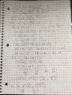# Trying to find two vectors with known sum, what am I doing wrong?

Joshua G
Homework Statement:
I'm trying to find two vectors whose sum is <2,0> ; where v_1 is parallel to <2,5> and v_2 is perpendicular to <2,5>.
Relevant Equations:
<v1>*<v2>=0 for orthogonal
<v1>*<v2>=1 for parallelv_1 = <-8/21,-20/21>
v_2 = <50/21,-20/21> When I take the dot product of v_2 and <2,5> I get zero, indicating they are perpendicular. Sorry for the hand writing.